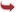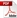International Journal of Scientific and Research Publications

#### IJSRP, Volume 4, Issue 5, May 2014 Edition [ISSN 2250-3153]Bifurcation Analysis and Fractal Dimensions of a Non-Linear Map
Ruma Saha
Abstract: In this paper a two dimensional non-linear map is taken, whose various dynamic behavior is analyzed. Some useful numerical algorithms to obtain fixed points and bifurcation values of period , . have been discussed. It has shown how the ratio of three successive period doubling bifurcation points ultimately converge to the Feigenbaum constant. This ascertains that the map follows the period doubling route to chaos. The parameter value where chaos starts is verified by lyapunov exponent. Further various fractal dimensions like Correlation dimension, Box-counting and Information dimension have been calculated to verify the geometry of the strange attractor.
[VIEW FULL PAPER][DOWNLOAD]

Reference this Research Paper (copy & paste below code):

Ruma Saha (2018); Bifurcation Analysis and Fractal Dimensions of a Non-Linear Map; Int J Sci Res Publ 4(5) (ISSN: 2250-3153). http://www.ijsrp.org/research-paper-0514.php?rp=P292657